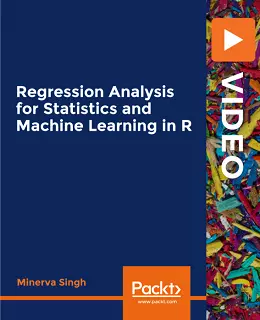# Regression Analysis for Statistics and Machine Learning in R [Video]### Regression Analysis for Statistics and Machine Learning in R [Video]

##### English | MP4 | AVC 1920×1080 | AAC 44KHz 2ch | 7h 18m | 1.28 GB eLearning | Skill level: All Levels

Regression Analysis for Statistics and Machine Learning in R [Video]: Learn complete hands-on Regression Analysis for practical Statistical Modelling and Machine Learning in R

With so many R Statistics and Machine Learning courses around, why enroll for this?

Become a Regression Analysis Expert and Harness the Power of R for Your Analysis:

• Get started with R and RStudio. Install these on your system, learn to load packages, and read in different types of data in R
• Carry out data cleaning and data visualization using R
• Implement Ordinary Least Square (OLS) regression in R and learn how to interpret the results.
• Learn how to deal with multicollinearity both through the variable selection and regularization techniques such as ridge regression
• Carry out variable and regression model selection using both statistical and machine learning techniques, including using cross-validation methods.
• Evaluate the regression model accuracy
• Implement Generalized Linear Models (GLMs) such as logistic regression and Poisson regression. Use logistic regression as a binary classifier to distinguish between male and female voices.
• Use non-parametric techniques such as Generalized Additive Models (GAMs) to work with non-linear and non-parametric data.
• Work with tree-based machine learning models

Regression analysis is one of the central aspects of both statistical- and machine learning-based analysis. This course will teach you regression analysis for both statistical data analysis and machine learning in R in a practical, hands-on way. It explores relevant concepts in a practical way, from basic to expert level. This course can help you achieve better grades, gain new analysis tools for your academic career, implement your knowledge in a work setting, and make business forecasting-related decisions. You will go all the way from implementing and inferring simple OLS (Ordinary Least Square) regression models to dealing with issues of multicollinearity in regression to machine learning-based regression models.